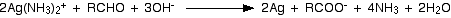# How can you find the weakest oxidising agent from the following? sf(Ag_2O, "acidified"color(white)(x)MnO_4^(-),Cl_2, "acidified"color(white)(x)Cr_2O_7^(2-))

Jun 17, 2015

$A {g}_{2} O$ would be considered a mild oxidising agent when compared to the other three.

#### Explanation:

The best way to compare the relative strength of oxidising agents is to use standard electrode potentials or ${E}^{\circ}$ values.

They should be listed most negative to most positive. A fairly comprehensive list can be found here:

The ones we need are:

$A {g}^{+} + e r i g h t \le f t h a r p \infty n s A g \ldots \ldots \ldots \ldots \ldots \ldots \ldots \ldots \ldots \ldots \ldots \ldots \ldots \ldots \ldots . . {E}^{\circ} = + 0.8 \text{V}$

$C {r}_{2} {O}_{7}^{2 -} + 14 {H}^{+} + 6 e r i g h t \le f t h a r p \infty n s 2 C {r}^{3 +} + 7 {H}_{2} O \ldots \ldots . . {E}^{\circ} = + 1.33 \text{V}$

$C {l}_{2} + 2 e r i g h t \le f t h a r p \infty n s 2 C {l}^{-} \ldots \ldots \ldots \ldots \ldots \ldots \ldots \ldots \ldots \ldots \ldots \ldots \ldots \ldots . . {E}^{\circ} = + 1.36 \text{V}$

$M n {O}_{4}^{-} + 8 {H}^{+} + 5 e r i g h t \le f t h a r p \infty n s M {n}^{2 +} + 4 {H}_{2} O \ldots \ldots \ldots \ldots {E}^{\circ} = + 1.51 \text{V}$

The most powerful oxidisers on this table are found at the bottom left.

These can, in theory, oxidise any species above them on the right if the activation energy is not too high.

Since $A {g}^{+}$ is at the top of the table we can consider it to be the weakest of the oxidising agents listed.

Please note these data refer to standard conditions. By altering the conditions we may be able to drive reactions in the direction we want.

The most common example by which $\text{Ag(I)}$ acts as an oxidiser is in the laboratory test for an alkanal, or aldehyde group $R C H O$.

Ammonia solution is added to aqueous $A {g}^{+}$ to form a precipitate of $A g O H$. When XS is added the diammine silver(I) complex is formed.

This is also known as "Tollen's Reagent". It is used to identify the presence of an alkanal (aldehyde) group.

It is able to oxidise an alkanal group to a carboxylic acid as follows:You see a "mirror" effect as silver is formed in the test tube hence it is known as the "silver mirror test".

It looks like this: